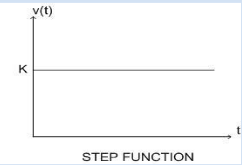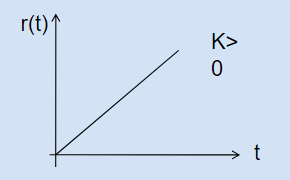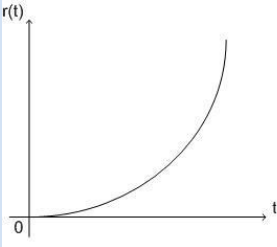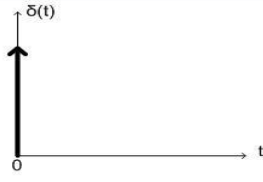### Test Signals for Time Response

Test Signals are the signals used to test the performance of the system using the time response analysis of the output. Following are some standard test signals that are used for time response analysis of a control system:

Step Function:
An unit step signal is constant for all positive values of time(t) inclusive of t=0. Step signal does not exist(or zero) for negative values of ‘t’.
Mathematically a step signal is represented as f(t),
Where
f(t)=k ; for t>=0 ,
f(t)=0 : for t<0Unit step signal is denoted by u(t), where k=1

Ramp Function:
A ramp function starts at (0,0) and increases linearly with time(t) with a constant slope. For a unit ramp signal the slope is unity.
Mathematically a ramp signal can be represented as r(t),
Where
r(t)=K.t ; for t>=0 (K is the constant slope)
r(t)=0 ; for t<0A ramp function can be written in terms of unit step signal as follows:
r(t)=K.t.u(t)

Parabolic function:
A parabolic function is a quadratic function of time(t) for positive values of ‘t’ while zero for negative values of t.
Mathematical representation of a parabolic signal:
p(t)=K ; for t>=0
p(t)=0 ; for t<0
For an unit parabolic signal the value of constant K is unity.A Ramp signal can be represented using a unit step signal as:
r(t) = Ku(t)

Unit Impulse Signal:
An unit impulse signal only exists for t=0 and is zero for all other values of time(t). The amplitude of this signal at t=0 tends to infinity such that the area of the function at the instant t=0 is unity. Impulse function is also known as delta function.
Mathematical representation:
; for t0
; for t=0